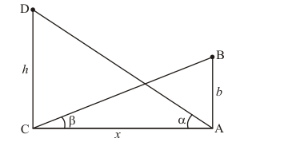# A tower subtends an angle α at a point A in the plane of its base

Question:

A tower subtends an angle α at a point A in the plane of its base and the angles of depression of the foot of the tower at a point b metres just above A is β. Prove that the height of the tower is b tan α cot β.

Solution:

Letbe the height of tower. The tower CD subtends an angleat a point. And the angle of depression of foot of tower at a point b meter just aboveis. Letand.

Here we have to prove height of tower isWe have the corresponding figure as followsSo we use trigonometric ratios.

In,

$\Rightarrow \quad \tan \beta=\frac{A B}{A C}$

$\Rightarrow \quad \tan \beta=\frac{b}{x}$

$\Rightarrow \quad x=\frac{b}{\tan \beta}$

$\Rightarrow \quad x=b \cot \beta$

Again in $\triangle A C D$

$\Rightarrow \quad \tan \alpha=\frac{C D}{A C}$

$\Rightarrow \quad \tan \alpha=\frac{h}{x}$

$\Rightarrow \quad h=x \tan \alpha$

$\Rightarrow \quad h=b \tan \alpha \cot \beta$

Hence the height of tower is $b \tan \alpha \cot \beta$.